# Some pictures of stochastic Julia sets

These objects are studied in my paper Stochastic equidistribution and Generalized Adelic Measures, joint with John Doyle and Bella Tobin.

Here you can find the slides from my talk at the conference "Algebraic Dynamics and its Connections to Difference and Differntial Equations" at BIRS in November 2021. We included in this talk several renderings of stochastic Julia sets. These were done with Sage, using the idea of taking random backwards orbits under the system.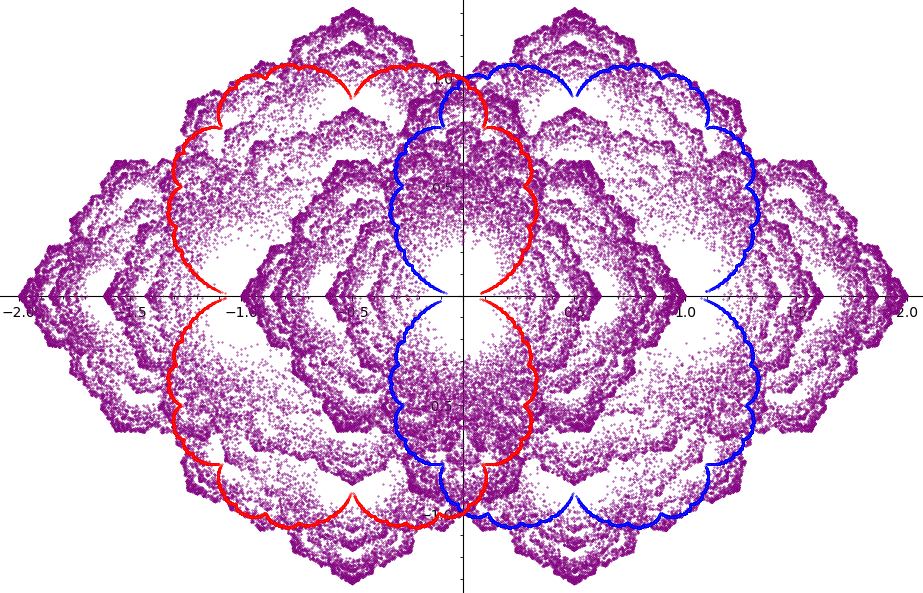Above: Picture of f(z) = z + z2 and g(z)=z - z2 with equal weight. Julia sets of f and g in red and blue, respectively, and the stochastic Julia set in purple.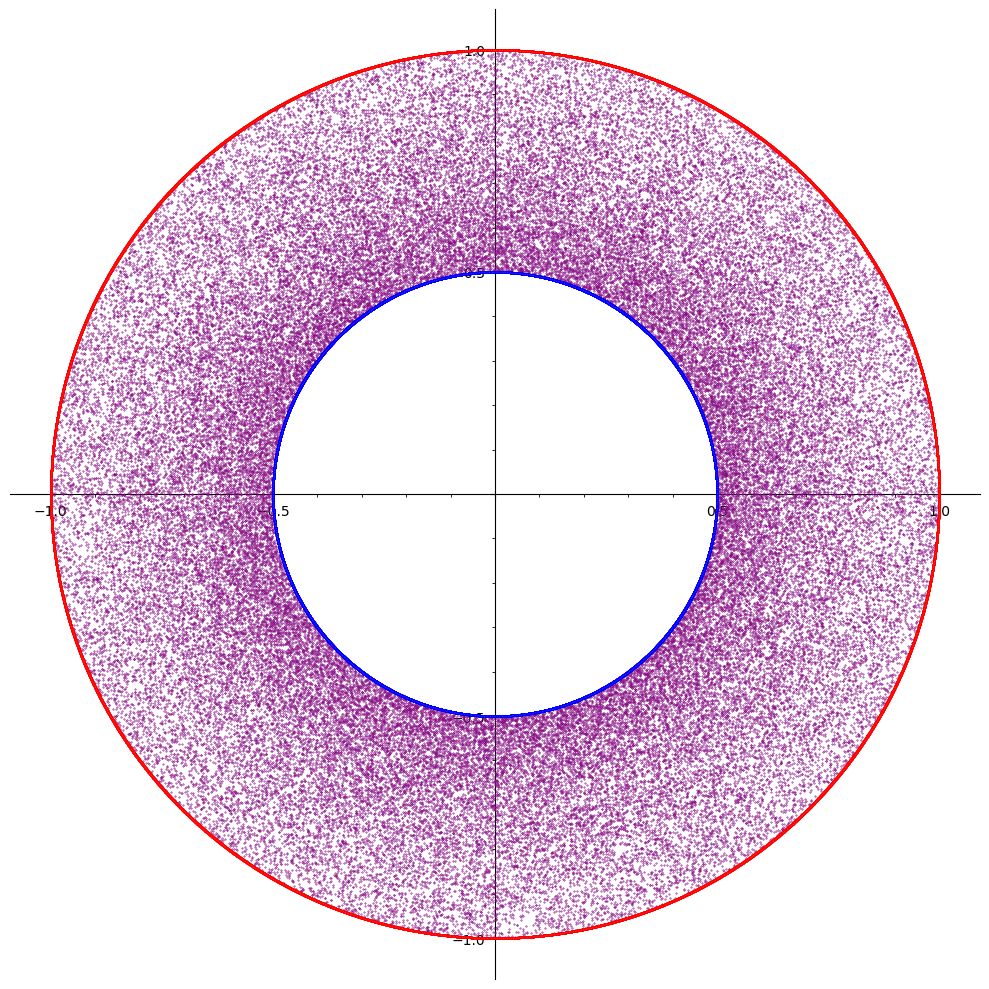Above: Picture of f(z) = z2 and g(z)=2z2 with equal weight. Julia sets of f and g in red and blue, respectively, and the stochastic Julia set in purple.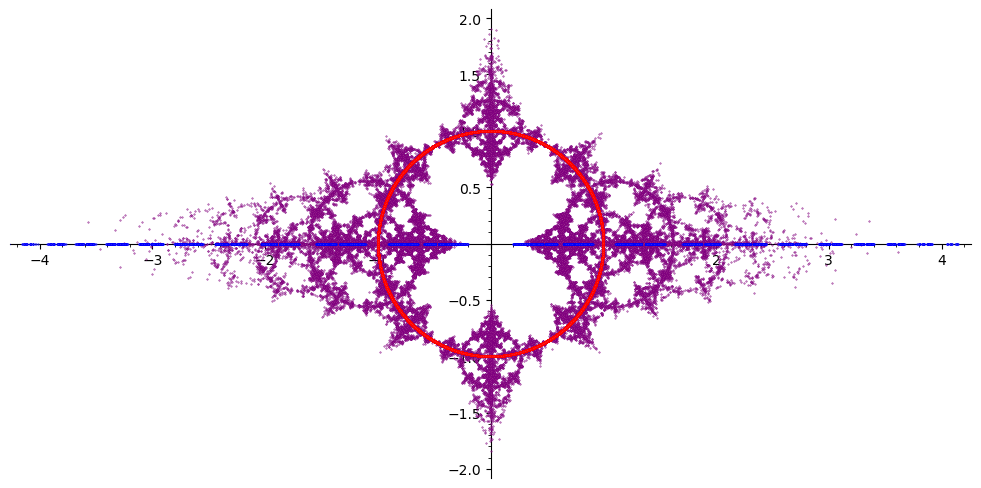Above: Picture of f(z) = z2 and g(z)=z - 1/z with equal weight. Julia sets of f and g in red and blue, respectively, and the stochastic Julia set in purple.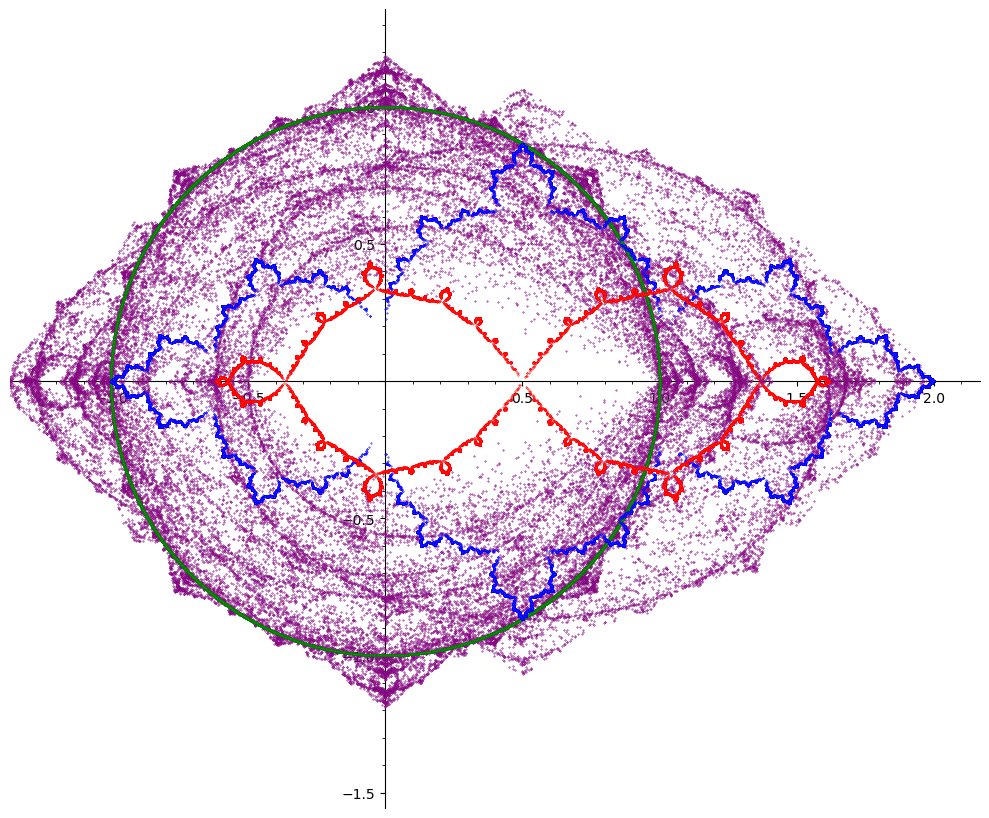Above: Picture of f(z) = -2z3 + 3z2, g(z)=z2 - z and h(z) = z2 with probabilities 0.1, 0.2, and 0.7, respectively. Julia sets of f, g and h in red, blue, and green, respectively, and the stochastic Julia set in purple.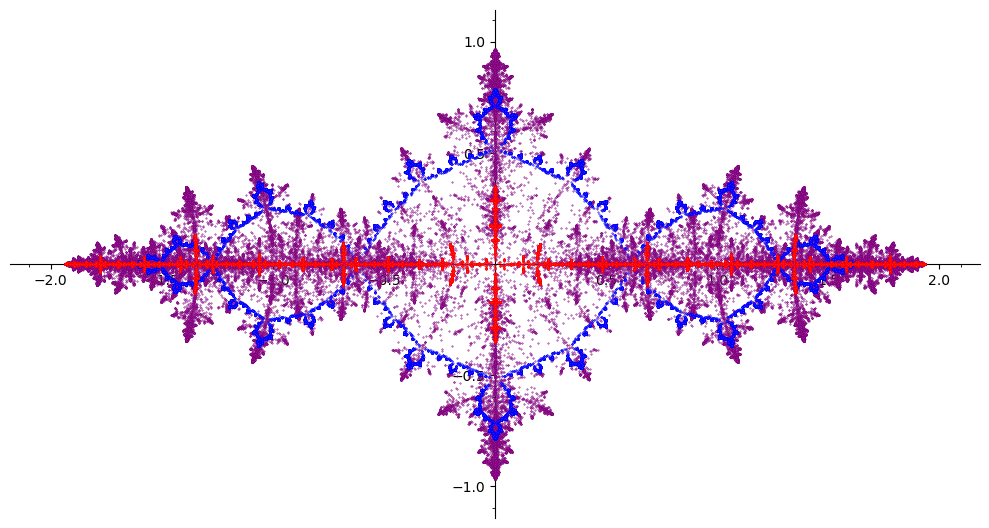Above: Picture of f(z) = z2 - 29/16 and g(z)=z2 - 1 with equal weight. Julia sets of f and g in red and blue, respectively, and the stochastic Julia set in purple.Above: Picture of f(z) = (2z - 1/3)2 + 1/3 and g(z)=z2 with equal weight. Julia sets of f and g in red and blue, respectively, and the stochastic Julia set in purple.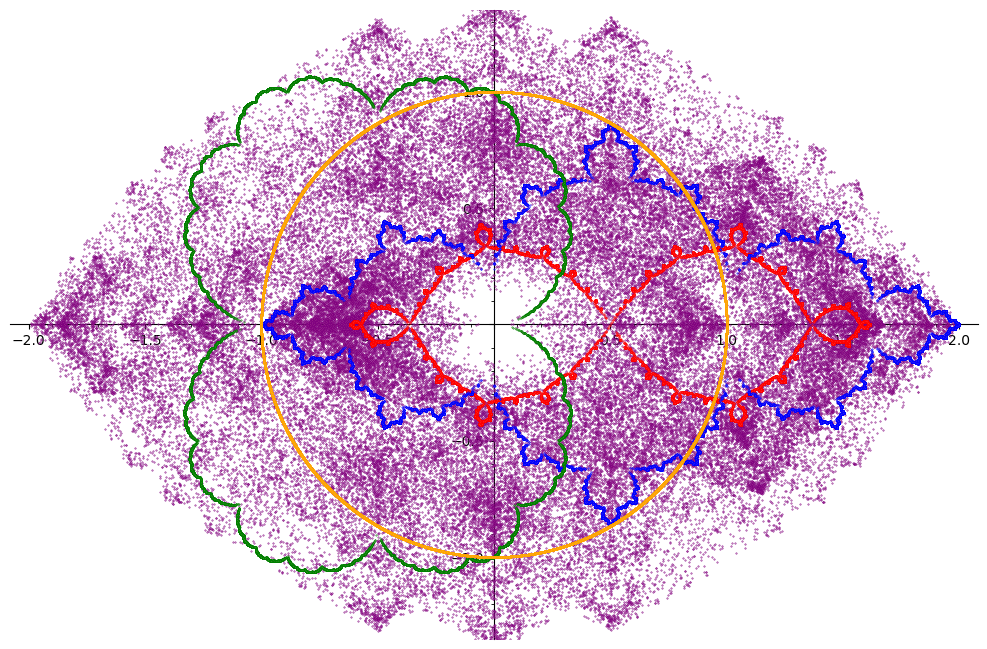Above: Picture of f(z) = 2z3 - 3z2 + 1, g(z)=z2 - z, u(z)=z2 + z, and v(z) = z2 with equal probabilities. Julia sets of f, g, u, and v in red, blue, green, and orange, respectively, and the stochastic Julia set in purple.

Last updated Nov. 24, 2021.